# Unrestricted General Solution of 6DoF Inverse Dynamics Problem of a 3D Guided Glider

Unrestricted General Solution of 6DoF Inverse Dynamics Problem of a 3D Guided Glider

Abdallah M. ElsherbinyAmgad M. Bayoumy Ahmed M. Elshabka Mohamed M. Abdelrahman

EAF Research and Development Department, MTC, Cairo 11766, Egypt

Mechatronics Department, MSA University, Giza 12563, Egypt

Aeronautical Department, MTC, Cairo 11766, Egypt

Aeronautical Department, Cairo University, Cairo 12613, Egypt

Corresponding Author Email:
ambayoumy@msa.eun.eg
Page:
465-475
|
DOI:
https://doi.org/10.18280/mmep.070318
24 June 2020
|
Accepted:
30 August 2020
|
Published:
30 Sptember 2020
| Citation

OPEN ACCESS

Abstract:

Although solving inverse dynamics problems is performed a lot in literature, none of the previous references addressed the general unrestricted solution of three dimensional (3D) trajectories of guided gliders. This glider could be any subsonic flying body such as guided ammunitions. Inverse dynamics could be one of the key techniques of solving similar problems. In this paper, 3D trajectory generation and following are performed. The trajectory generation is divided into three phases: heading correction, glide and terminal phases. The solution of the inverse problem is performed analytically, using the Mu-Pad tool. Next, a special formulation of the general dynamics equations enables the solution of such a problem and the calculation of the required deflection angles. Finally, a six degrees of freedom (6DoF) direct simulation is performed using the obtained deflection angles in order to compare its trajectory with the generated one. This comparison yields fairly good results and validates the quality of the proposed inverse dynamics solution.

Keywords:

inverse simulation, direct simulation, trajectory generation, guided glider

1. Introduction

The trajectory generation and following are very critical for most unmanned aerial vehicles and guided weapons which mainly depend on GPS/INS systems for location detection. The trajectory generation can be applied for different types of flying vehicles such as unmanned combat air vehicles (UCAV) , entry vehicles , UAVs with obstacle avoidance mechanisms , missiles , guided projectiles , and guided gliding vehicles [6, 7].

The guided gliders are discussed here because many types of aerial vehicles could be considered as gliders in the case of emergencies (such as when the engine is down).

Regarding the trajectory generation, the trajectory is divided into gliding and terminal phases. There are many approaches for obtaining the optimum gliding trajectories depending on the mission requirements, such as maximum range [8, 9] or maximum endurance . These problems can be solved using indirect and direct methods. The indirect methods transform a control problem into a boundary value problem which is very accurate but also very complicated . Many studies concerned with the indirect methods use the inverse simulation problem to obtain the optimum trajectory [4, 12-14]. On the other hand, studies using the direct methods obtain the optimum trajectory through the nonlinear programming problem (NLP) [15, 16].

For the terminal phase, the problem is to obtain the maximum impact velocity and angle which can be solved using the searching methods  or initial parameterization problem methods. Furthermore, the initial parameterization problem can be solved using indirect  or direct methods [5, 19, 20].

The inverse dynamics technique is performed to determine the time history of the control inputs that enable a dynamic system to follow a certain desired trajectory or behavior. The inverse dynamics problem can be classified as three degrees of freedom (3DoF) and six degrees of freedom (6DoF). For the 3DoF, a point mass aircraft model is applied to control the longitudinal motion [21-23] or the roll motion .

Few studies introduce 6DoF inverse simulations. In 1994, Abdelrahman and Al-Bahi  introduced a generalized technique for the inverse simulation of aircraft motion along predetermined trajectories. The inputs of the inverse simulations are the three components of the trajectory, as well as the bank angle command [x(t), y(t), z(t), ϕ(t)]. The simulations applied in their work are divided into simulation of vertical plane motion, horizontal loop maneuver and rolling maneuver; separately. In each simulation, they made assumptions to obtain the differential equations and the solution procedures. In 2002, Blajer et al.  introduced an inverse simulation study of an aircraft flight path reconstruction. They also made two assumptions in their trajectory that the altitude and velocity are constants, which decreases the number of variables in the governing equations. In 2016, Yang and Yan  introduced a “Neural Network Approximation-based Nonsingular Terminal Sliding Mode Control Approach” for solving the issues of the trajectory following for robotic air vehicles. The inputs are six components of the trajectory and attitudes as functions of time [x(t), y(t), z(t), ϕ(t), θ(t), ψ(t)]. Accordingly, the attitudes must be specified before applying this inverse simulation technique.

In 2019, Yang et al. introduce a dual quaternion approach for solving the 6DoF inverse simulation of a parallel robots. This approach decreases the computational cost and time besides avoiding the singularities. They avoid using the Euler’s angle approach because of its interminable trigonometric functions which leads to a higher computational cost .

As concluded by the above survey, there is no general unrestricted technique to solve the 6DoF, position and Euler angles, inverse dynamics problem of a guided glider over a 3D trajectory. In this paper, a new nonlinear 6DoF generalized inverse simulation technique is proposed and applied on the 3D generated trajectory of a guided glider flying body.

To overcome the computational cost of multiple trigonometric functions, pre-differntionation formulas of the inverse dynamics equations of motion are derived symbolically which can be solved as algebraic equations where the cosines and sines of the Euler angles are calculated once for each time step.

The inputs of this method are the trajectory and flight bank angle as functions of trajectory length [x(s), y(s), z(s), μ(s)]. The outputs of this method are the velocity components, the attitudes, the angular rates, and the three deflection angles [Vt, α, β, ϕ, θ, ψ, P, Q, R, δp, δr, δy]. Furthermore, a validation of the inverse simulation technique is applied by comparing its response and trajectory with a developed 6DoF direct simulation that uses the calculated control deflection angles [δp, δr, δy] as inputs. The flying body dimensions and data are pre-calculated using a hybrid optimization aerodynamic design .

2. Trajectory Generation

The trajectory of the flying body is planned depending on the mission requirements. For the problem in hand, the mission requires achieving maximum target destruction while maintaining the distance between the release-point and the target-location as large as possible. As a result, the trajectory’s range, impact angle and speed should be maximized. When the trajectory is generated with a maximum lift-to-drag ratio, it will necessarily lead to a maximum glide range but will also result in a minimum impact angle and speed (and vice-versa). For this reason, the trajectory is divided into three phases. Each of these phases uses the appropriate optimization function that fulfills its specific requirements. The three phases of the 3D trajectory generation are: the heading correction, the direct glide, and the terminal phases. The idea of the 3D trajectory optimization is to apply a nonlinear programing method that depends on the harp angle (shown in Figure 1) as a design parameter to transform the trajectory from the direct glide phase into the terminal phase.

## 1.pngFigure 1. The line of sight and the harp angle 

The harp angle is the one whose tangent is equal to the release altitude divided by the range . In order to smooth the transition in the 3D trajectory between phases, a Bézier quadratic curve technique is applied.

The heading correction phase is needed when the flying body is released with an offset heading angle to the target. This happens when the vertical plane of the flying body does not coincide with the line-of-sight’s vertical plane. The line of sight's vertical plane is the plane formed by the range and the slant range lines.  In this case, the flying body will need to perform a horizontal turn during the glide until it reaches the vertical plane of the line-of-sight achieving a zero heading-offset angle.

To achieve this horizontal turn, the flying body should perform two turns. The first turn enables the flying body to reach the target vertical plane quickly. To achieve that, this turn is performed with minimum radius using Eqns. (1) and (2). The second turn has a very large curvature since it is concerned with enabling the flying body’s vertical plane to coincide with the target vertical plane in a very smooth manner. Figure 2 shows the horizontal and vertical projections of the first turn of the heading correction phase.

${{x}_{turn\text{ }glid{{e}_{i}}}}=\frac{{{H}_{\text{s}tart}}-{{H}_{turn\text{ }glid{{e}_{i}}}}}{\tan \gamma }$      (1)

${{y}_{turn\text{ glid}{{\text{e}}_{i}}}}=-\left( \frac{2{{R}_{\text{t}urn}}-\sqrt{4{{R}_{\text{turn}}}^{2}+4{{x}_{turn\text{ glid}{{\text{e}}_{i}}^{2}}}^{{}}}}{2} \right)$     (2)

## 2.png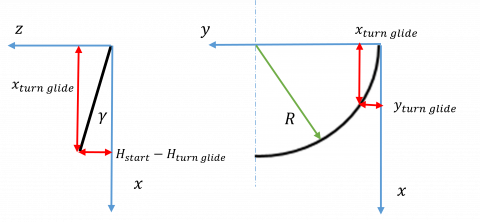Figure 2. The heading correction turn dimensions

## 3.pngFigure 3. The heading correction phase

The smoothing turn is performed using a Bézier quadratic interpolation given by Eq. (3) using three control points as shown in Figure 3. The first point P1 is selected at the end of the first turn’s curve. The second point P2 is selected to be the intersection point of the tangent to the first turn’s curve and the line of sight's vertical plane. Finally, the third point P3 is selected as a point lying on the target vertical plane after the double distance between the first and the second points to ensure a smooth curve.

$P_{i}(x, y, z)=\left(1-t d_{i}\right)^{2} P_{1}(x, y, z)$$+2 t d_{i}\left(1-t d_{i}\right) P_{2}(x, y, z)$$+\left(t d_{i}\right)^{2} P_{3}(x, y, z)$     (3)

The glide phase trajectory is generated with a minimum glide angle to ensure a maximum lift-to-drag ratio using Eqns. (4), (5), and (6). The glide phase ends when the flying body reaches the point (P4) at which the harp angle (qz) equals its optimum value as given in Eq. (7) to match that of the terminal phase.

2.2 Glide phase

$x_{\text {glide }}=\left(\frac{H_{\text {glide start }}-H_{i}}{\tan \gamma}\right) * \cos \psi_{\text {of f set }}$      (4)

$y_{\text {glide }}=\left(\frac{H_{\text {glide start }}-H_{i}}{\tan \gamma}\right) * \sin \psi_{\text {of fset }}$    (5)

$\psi_{\text {offset}_{i}}=\tan ^{-1} \frac{y_{\text {target}}-y_{i}}{x_{\text {target}}-x_{i}}$          (6)

$q_{z}=\tan ^{-1}\left(\frac{H_{i}-H_{\text {target}}}{\sqrt{x^{2} \operatorname{target}+y^{2} \operatorname{target}}-\sqrt{x^{2} i+y^{2}}_{i}}\right)$    (7)

The optimization problem can be described as:The optimization problem is performed by minimizing the flight path angle as an objective function. This optimization is achieved through a 6DoF direct simulation.

The optimization problem can be described as:

$\min f\left(X_{1}\right)=\gamma$

$-4 \leq \alpha \leq 8$

subject to:     $q_{z} \leq 35$

$X_{1}=[\alpha]$

2.3 Terminal phase

The starting point of the terminal phase is obtained using a direct method as an initial parameterization problem solved by nonlinear programming (NLP). The gradient-based optimization method is used to solve the NLP problem with the objective function being the impact velocity. The constraints are the angle of attack and the impact angle, while the design parameters are the harp angle and the flight path angle at the impact point. This optimization problem can be described as:

$\max f\left(X_{2}\right)=V_{ {impact}}$

$-4 \leq \alpha \leq 8$

subject to:      $75 \leq \theta_{{impact}} \leq 89$

$X_{2}=\left[\begin{array}{ll}q_{z} & \gamma_{ {impact}}\end{array}\right]$

The harp angle and the flight path angle at the impact point are found to be 35˚ and 78˚ respectively. The terminal phase trajectory can be drawn in (x, y, z) coordinates using the Bézier quadratic spline of three control points as shown in Figure 4. The first point P4 is at the end of the glide phase; obtained previously. The second point P5 is the intersection of the impact angle and glide angle lines, and the third point P6 is the given target position. Apparently, point P5 is the only unknown point in these three points. Next, the second point coordinates (xp5, yp5, zp5) could be obtained using the Eqns. (8) to (10). In Eq. (8) and Eq. (9), γ is the flight path angle of the glide phase which is known, γimpact is the flight path angle at the impact point which is known from the optimization output. So, the unknowns are (xp5, yp5, zp5).

In Eq. (10), ψoffset is the offset angle of the target from release point which is known previously and yp5 can be substituted as function of xp5 in Eqns. (8, 9). Consequently, Eqns. (8, 9) can be solved to calculate [xp5, zp5,], then equation (10) to calculate yp5.

$\frac{z_{p 4}-z_{p 5}}{\sqrt{x_{p 5}^{2}+y_{p 5}^{2}}-\sqrt{x_{p 4}^{2}+y_{p 4}^{2}}}=\tan \gamma$        (8)

$\frac{z_{p 5}-z_{p 6}}{\sqrt{x_{p 6}^{2}+y_{p 6}^{2}}-\sqrt{x_{p 5}^{2}+y_{p 5}^{2}}}=\tan \gamma_{i m p a c t}$       (9)

$x_{p i} \tan \psi_{\text {offset}}=y_{p i}, \quad i \in\{4,5,6\}$     (10)

The Bézier curve shown in Figure 4 is generated from one hundred intermediate points in [x, y, z] coordinates that are calculated using Eq. (3). The flight path angle during the terminal phase can be calculated at each step using Eq. (11). It is found that the optimum harp angle is equal to 35˚.

$\gamma_{\text {diving phase}}=\tan ^{-1} \frac{-\Delta z}{\Delta x y}$       (11)

## 4.pngFigure 4. Generated trajectory of terminal phase showing the control points

2.4 Overall trajectory

The overall trajectory is obtained by combining the arbitrary data points [x, y, z] of the three phases as shown in Figure 5. First, the flying body is released in the airplane’s vertical plane. It then performs a gliding turn to correct its heading and continues gliding till the harp angle reaches its optimum value. Finally, it performs a vertical turn to reach the target.

## 5.png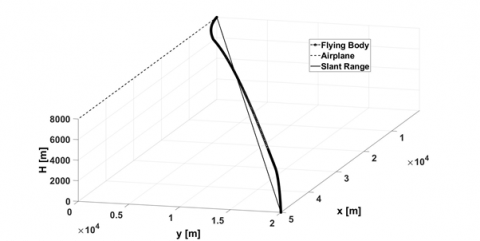Figure 5. Slant range, airplane, and overall trajectory

3. Inverse Simulation

After generating the optimum trajectories, inverse simulations are carried out to arrive at the control surface deflection angles. The direct simulation model includes twelve flying body equations of motion (Eqns. (12-23)). If the three control surfaces' deflection angles U(t) and the initial values of the state vector Xi are given, the twelve variables of the state vector X(t), including the flight trajectory [x(t), y(t), z(t)] and the flight attitudes [ϕ(t), θ(t), ψ(t))], can be obtained. However, in the inverse dynamics problem if the state vector X(t) is used as an input, there will be no solution. This is a result of having twelve inputs (flying body states) and three outputs, [δp(t), δr(t), δy(t)] . Furthermore, if the inputs of the state vector are less than three, there will be infinite solutions. Therefore, the inverse simulation is performed by using only three inputs of the state vector to obtain a single solution of the control vector U(t). In this study, a guided glider flying body is considered. Hence, the thrust is zero. In addition, the generated trajectories are arbitrary points given in (x, y, z) coordinates as a function of the flight path length(s). Additionally, new nonlinear generalized 6DoF inverse simulations are performed using both flight path and body equations of motion.

The general inverse simulation equations of motion of a flying body can be presented in the flight path axes  as follows:

$\dot{x}=V \cos \gamma \cos \chi$    (12)

$\dot{y}=V \cos \gamma \sin \chi$      (13)

$\dot{z}=-V \quad \sin \gamma$       (14)

$-D-m g \sin \gamma-m \dot{V}=0$    (15)

$-Y+m g \sin \mu \cos \gamma$$+m V(-\dot{\chi} \cos \mu \cos \gamma$$+\dot{\gamma} \sin \mu)=0$                (16)

$L-m g \cos \mu \cos \gamma$$-m V(\dot{\chi} \sin \mu \cos \gamma+\dot{\gamma} \cos \mu)$$=0$         (17)

$\dot{\phi}=P+(Q \sin \phi+R \cos \phi) \tan \theta$    (18)

$\theta=Q \cos \phi-R \sin \phi$      (19)

$\psi=(Q \sin \phi+R \cos \phi) / \cos \theta$     (20)

$\dot{P}=\left(C_{1} R+C_{2} P\right) Q+C_{3} \bar{L}+C_{4} N$    (21)

$\dot{Q}=C_{\mathrm{S}} P R-C_{\mathrm{f}}\left(P^{2}-R^{2}\right)+C_{7} M$   (22)

$\dot{P}=\left(C_{8} P-C_{2} R\right) Q+C_{4} \bar{L}+C_{9} N$    (23)

The general inverse simulation of a flying body has nine equations (three kinematics equations and six dynamics equations) with twelve unknowns. Hence, three constraint equations must be added. The inputs are the arbitrary trajectory data (x(s), y(s), z(s), μ(s)) that can be transformed into the flight path angles (γ(s), χ(s), μ(s)). The outputs are the control surfaces' deflections (δpitch, δyaw, δroll) that are transformed into the fins' deflection angles (δ1, δ2, δ3, δ4).

The difficulty of formulating a general inverse simulation problem lies in the determination of the first and second derivatives of the three attitudes ($\dot{\phi}, \dot{\theta}, \dot{\psi}, \ddot{\phi}, \ddot{\theta}, \ddot{\psi}$) without any further restrictions. The body axes and flight path axes are related by the angle of attack (α) and the sideslip angle (β) . The rotation from the flight path axes to the body axes can be performed using the following equations, (24) to (27).

$R(\phi, \theta, \psi)=R_{1}(-\beta, \alpha, 0) \cdot R_{2}(\mu, \gamma, \chi)$         (24)

$R_{1}(-\beta, \alpha, 0) =\left[\begin{array}{ccc}\cos \beta \cos \alpha & -\sin \beta \cos \alpha & -\sin \alpha \\ \sin \beta & \cos \beta & 0 \\ \cos \beta \sin \alpha & -\sin \beta \sin \alpha & \cos \alpha\end{array}\right]$      (25)

$R_{2}(\mu, \gamma, \chi)=\left[\begin{array}{ccc}\cos \chi \cos \gamma & \sin \chi \cos \gamma & -\sin \gamma \\ \cos \chi \sin \gamma \sin \mu-\sin \chi \cos \mu & \sin \chi \sin \gamma \sin \mu+\cos \chi \cos \mu & \cos \gamma \sin \mu \\ \cos \chi \sin \gamma \cos \mu+\sin \chi \sin \mu & \sin \chi \sin \gamma \cos \mu-\cos \chi \sin \mu & \cos \gamma \cos \mu\end{array}\right]$ (26)

$R(\phi, \theta, \psi)$$=\left[\begin{array}{ccc}\cos \psi \cos \theta & \sin \psi \cos \theta & -\sin \theta \\ \cos \psi \sin \theta \sin \phi-\sin \psi \cos \phi & \sin \psi \sin \theta \sin \phi+\cos \psi \cos \phi & \cos \theta \sin \phi \\ \cos \psi \sin \theta \cos \phi+\sin \psi \sin \phi & \sin \psi \sin \theta \cos \phi-\cos \psi \sin \phi & \cos \theta \cos \phi\end{array}\right] (27) In order to formulate the pitch angle (θ), presented in Eq. (28), the element R1,3 must be obtained from Eq. (27). \theta=\sin ^{-1}[\cos \alpha \cos \beta \sin \gamma+\cos \gamma \cos \mu \sin \alpha$$+\cos \alpha \cos \gamma \sin \beta \sin \mu]$          (28)

Similarly, the other Euler angles (ϕ, ψ) are calculated using the elements R2,3 and R1,2 respectively and are shown in Eqns. (29) and (30):

$\phi=\sin ^{-1}[-(\sin \beta \sin \gamma+\cos \beta \cos \gamma \sin \mu) / \cos \theta]$      (29)

$\psi=\sin ^{-1}[(\sin \alpha(\cos \chi \sin \mu-\cos \mu \sin \gamma \sin \chi)$$-\cos \alpha \sin \beta(\cos \chi \cos \mu$$+\sin \gamma \sin \chi \sin \mu)$$+\cos \alpha \cos \beta \cos \gamma \sin \chi) / \cos \theta] (30) Moreover, by substituting the value of (θ) from equation (28) in Eqns. (29) and (30), the body attitudes (ϕ, θ, ψ) are obtained as functions of the flight path angles (μ, γ, χ) and wind angles (β, α) only. Consequently, the derivatives (\dot{\phi} . \dot{\theta} . \dot{\psi} . \ddot{\phi} . \ddot{\theta} . \ddot{\psi}) can be obtained by differentiating the body attitudes twice with respect to time. Differentiating the three trigonometric Eqns. (28), (29) and (30) is greatly challenging owing to its complexity. For this reason, previous studies usually avoid this method. However, the methodology of differentiating the three equations plays a vital role in ensuring the accuracy of the resulting equations. As a result, a program is developed in the current study to perform the symbolic differentiation of the three equations using MuPAD/MATLAB and the resulting equations were then imported into the main MATLAB program. The six resulting equations of attitude derivatives are lengthy, hence they are presented in Appendix A. The following parameters are necessary to perform the 6DoF inverse simulation: Ⅰ. Attitudes and their derivatives up to the second derivatives (Appendix A) Ⅱ. Flight path angles and their derivatives up to the third derivatives (\gamma, \chi, \mu, \dot{\gamma}, \ddot{\gamma}, \dddot{\gamma}, \dot{\chi}, \ddot{\chi}, \dddot{\chi}, \dot{\mu}, \ddot{\mu}, \dddot{\mu}) (Eqns. (33) to (43) ) Ⅲ. Velocity derivatives (\dot{V}, \ddot{V}) (Eqns. (15) and (44)). Ⅳ. Wind angles derivatives(\dot\alpha, \dot{\beta}, \ddot{\alpha}, \ddot{\beta}) (Eqns. (45) to (48)) Ⅴ. Angular rates derivatives (\dot{P}, \dot{Q}, \dot{R}) (Eqns. (49) to (51)) The equations from (33) to (51) represent the aerodynamic forces and moments, and demonstrate the technique of obtaining these required derivatives. They are also used to obtain the control surface deflection angles starting from the given trajectory data. The simulation is performed using the Runge-Kutta-4th order numerical integration method to obtain all variables of the state vector, X=[x, y, z, α, β, V, ϕ, θ, ψ, P, Q, R] at each time step. L=0.5 \rho V_{t}^{2} S\left[C_{L o}+C_{L \alpha} \alpha+C_{L \delta p} \delta_{p}\right.$$\left.+C_{L q}\left(c / 2 V_{T}\right) q+C_{L \dot{\alpha}}\left(c / 2 V_{T}\right) \dot{\alpha}\right]$

$D=0.5 \rho V_{t}^{2} S\left[C_{D o}+k C_{L}^{2}\right]$     (31)

$M=0.5 \rho V_{t}^{2} S c_{w}\left[C_{m o}+C_{m \alpha} \alpha+C_{m \delta_{p}} \delta_{p}\right.$$\left.+C_{m q}\left(c / 2 V_{T}\right) q+C_{m \dot{\alpha}}\left(c / 2 V_{T}\right) \dot{\alpha}\right] Y=0.5 \rho V_{t}^{2} S\left[C_{Y o}+C_{Y \beta} \beta+C_{Y \delta_{r}} \delta_{r}+C_{Y \delta_{y}} \delta_{y}\right.$$\left.+C_{Y p}\left(b / 2 V_{T}\right) p+C_{Y r}\left(b / 2 V_{T}\right) r\right]$

$\underline{L}=0.5 \rho V_{t}^{2} S b_{w}\left[C_{l o}+C_{l \beta} \beta+C_{l \delta r} \delta_{r}++C_{Y \delta y} \delta_{y}\right.$$\left.+C_{l p}\left(b / 2 V_{T}\right) p+C_{l r}\left(b / 2 V_{T}\right) r\right] (32) N=0.5 \rho V_{t}^{2} S b_{w}\left[C_{n o}+C_{n \beta} \beta+C_{n \delta_{r}} \delta_{r}+C_{Y \delta_{y}} \delta_{y}\right.$$\left.+C_{n p}\left(b / 2 V_{T}\right) p+C_{n r}\left(b / 2 V_{T}\right) r\right]$

3.1 Initial values calculation

1) Calculate $\gamma, \chi$ (Eqns. (33) and (34))

2) Calculate $\dot{\gamma}, \dot{\chi}, \dot{\mu}$ (Eqns. (35), (38), and (41))

3) Calculate $\alpha, \beta$ ((16) and (17))

4) Calculate $\theta, \psi, \phi$

5) Calculate $\dot{V}$ (Eq(15))

6) Calculate $\dot\beta, \dot{\alpha} (Eqns. (45) and (47)) 7) Calculate$\dot{\theta}, \dot{\psi}, \dot\phi$8) Calculate$P, Q, R$(Eqns. (18) to (20)$)$9) Calculate$\ddot V$(Eq.(44)) 10) Calculate$\ddot{\gamma}, \ddot{\chi}, \ddot{\mu}$(Eqns. (36), (39) and (42)) 11) Calculate$\dddot{\gamma}, \dddot{\chi}, \dddot{\mu}$(Eqns. (37), (40) and (43)) 12) Calculate$\ddot{\beta}, \ddot{\alpha}$(Eqns. (46) and (48)$)$13) Calculate$\ddot{\theta}, \ddot{\psi}, \ddot{\phi}$14) Calculate$\dot{P}, \dot{Q}, \dot{R}$(Eqns. (49) to (51)) 15) Calculate$M, N, L$(Eqns. (21) to (23)$)$16) Calculate$\delta_{\text {pitch }}, \delta_{\text {yaw }}, \delta_{\text {roll }}$(Eqns. (30) and (31)) 3.2 Numerical solution Starting with the initial values calculated in the above steps, the derivatives of all variables with respect to time are calculated as follows: 1) Calculate$\dot{x}, \dot{y}, \dot{z}$(Eqns. (12) to (14)) 2) Calculate$\dot{V}$(Eq. (15)) 3) Calculate$\dot{\gamma} \cdot \dot{\chi} \cdot \dot{\mu}($Eqns. (35),(38) and (41)$)$4) Calculate$\dot{\alpha}, \dot{\beta}$(Eqns. (45) and (47)$)$5) Calculate$\dot{\theta}, \dot{\psi}, \dot{\phi}$6) Calculate$\ddot{V}$(Eq. (44)) 7) Calculate$\ddot{\gamma}, \ddot{\chi}, \ddot{\mu}$Eqns. (36),(39) and (42)) 8) Calculate$\dddot{y}, \dddot{x}, \dddot{\mu}$(Eqns. (37), (40) and (43)) 9) Calculate$\ddot{\beta}, \ddot{\alpha}$(Eqns. (46) and (48)$)$10) Calculate$\ddot{\theta}, \ddot{\psi}, \ddot{\phi}$11) Calculate$\dot{P}, \dot{Q}, \dot{R}$(Eqns. (49) to (51)) 12) Calculate$M, N, L$(Eqns. (21) to (23)) 13) Calculate$\delta_{\text {pirch }}, \delta_{\text {yaw }}, \delta_{\text {roul }}$(Eqns. (30) and (31)). 14) Transfer$\delta_{\text {pitch }}, \delta_{\text {yaw },}, \delta_{\text {roll }}$into$\delta_{1}, \delta_{2}, \delta_{3}, \delta_{4}\gamma(s)=-\sin ^{-1}\left(z^{\prime}(s)\right)$(33)$\chi(s)=\tan ^{-1}\left(y^{\prime}(s) / x^{\prime}(s)\right)$(34)$\dot{\gamma}=\frac{d \gamma}{d t}=\frac{d \gamma}{d s} \frac{d s}{d t}\dot{\gamma}=\gamma^{\prime} V$(35)$\ddot{\gamma}=\frac{d \dot{\gamma}}{d t}=\frac{d}{d t}\left[\gamma^{\prime} V\right]=\frac{d \gamma^{\prime}}{d t} V+\frac{d V}{d t} \gamma^{\prime}=\frac{d \gamma^{\prime}}{d s} \frac{d s}{d t} V+\frac{d V}{d t} \gamma^{\prime}\ddot{\gamma}=\gamma^{\prime \prime} V^{2}+\gamma^{\prime} \dot{V}$(36)$\ddot{\gamma}=\gamma^{\prime \prime} V^{2}+\gamma^{\prime} \dot{V}\dddot{y}=\gamma^{\prime \prime \prime} V^{3}+2 \gamma^{\prime \prime} V \dot{V}+\gamma^{\prime \prime} V \dot{V}+\gamma^{\prime} \ddot{V}$(37) Similarly, the derivatives ($\dot{\chi}, \ddot{\chi}, \dddot{\chi}, \dot{\mu}, \ddot{\mu}, \dddot{\mu}$) are obtained.$\dot{x}=\chi^{\prime} V$(38)$\ddot{x}=\chi^{\prime \prime} V^{2}+\chi^{\prime} \dot{V}$(39)$\dddot{x}=\chi^{\prime \prime \prime} V^{3}+2 \chi^{\prime \prime} V \dot{V}+\chi^{\prime \prime} V \dot{V}+\chi^{\prime} \ddot{V}$(40)$\dot{\mu}=\mu^{\prime} V$(41)$\ddot{\mu}=\mu^{\prime \prime} V^{2}+\mu^{\prime} \dot{V}$(42)$\dddot{\mu}=\mu^{\prime \prime \prime} V^{3}+2 \mu^{\prime \prime} V \dot{V}+\mu^{\prime \prime} V \dot{V}+\mu^{\prime} \ddot{V}$(43) Differentiate Eq. (15) with respect to time to obtain \ddot{V}.$\begin{aligned} \ddot{V}=-\left[0.5 \rho S V^{2}\right.&\left(C_{D \alpha} \dot{\alpha}+C_{D \beta} \dot{\beta}\right)+0.5 \dot{\rho} S V^{2} C_{D} \\ &\left.+\rho S V \dot{V} C_{D}+m g \dot{\gamma} \cos \gamma\right] / m \end{aligned}$(44) Obtain \dot{\beta}, \ddot{\beta} by differentiating Eq. (16) twice with respect to time.$\begin{aligned} \dot{\beta}=[m V(\ddot{\gamma} \sin \mu-\ddot{\chi} \cos \gamma \cos \mu+\dot{\gamma} \dot{\mu} \cos \mu& \\+\dot{\chi} \dot{\gamma} \cos \mu \sin \gamma & \\+\dot{\chi} \dot{\mu} \cos \gamma \sin \mu) & m \dot{V}(-\dot{\chi} \cos \mu \cos \gamma\\+\dot{\gamma} \sin \mu)-0.5 \dot{\rho} S V^{2} C_{Y} &=\rho S V \dot{V} C_{Y} \\ &+m g(\dot{\mu} \cos \gamma \cos \mu\\ &-\dot{\gamma} \sin \gamma \sin \mu)] \\ & /\left(0.5 \rho S V^{2} C_{Y \beta}\right) \end{aligned}$(45)$\ddot{\beta}=\left\lceil m V\left(\dddot{\gamma} \sin \mu-\dot{\gamma} \dot{\mu}^{2} \sin \mu-\ddot{\chi} \cos \gamma \cos \mu\right.\right. \\ +\dot{\gamma} \ddot{\mu} \cos \mu+2 \dot{\mu} \ddot{\gamma} \cos \mu \\ +\dot{\chi} \dot{\gamma}^{2} \cos \gamma \cos \mu+\dot{\chi} \dot{\mu}^{2} \cos \gamma \cos \mu \\ +2 \dot{\gamma} \ddot{\chi} \cos \mu \sin \gamma+\dot{\chi} \ddot{\gamma} \cos \mu \sin \gamma+\dot{\chi} \ddot{\mu} \cos \gamma \sin \mu+2 \dot{\mu} \ddot{\chi} \cos \gamma \sin \mu \\ -2 \dot{\gamma} \dot{\chi} \dot{\mu} \sin \gamma \sin \mu) \\ +m \ddot{V}(-\dot{\chi} \cos \mu \cos \gamma+\dot{\gamma} \sin \mu)+2 m \dot{V}(\ddot{\gamma} \sin \mu-\ddot{\chi} \cos \gamma \cos \mu \\ +\dot{\gamma} \dot{\mu} \cos \mu+\dot{\chi} \dot{\gamma} \cos \mu \sin \gamma \\ +\dot{\chi} \dot{\mu} \cos \gamma \sin \mu)-\rho \dot{V}^{2} S C_{Y}-0.5 \ddot{\rho} S V^{2} C_{Y}-\rho S V \ddot{V} C_{Y} \\ -2 \dot{\rho} S V \dot{V} C_{Y}-\dot{\rho} S V^{2} \dot{\beta} C_{Y \beta} \\ -2 \rho S V \dot{V} \dot{\beta} C_{Y \beta}-m g \dot{\gamma}^{2} \cos \gamma \sin \mu \\ -m g \dot{\mu}^{2} \cos \gamma \sin \mu \\ +m g \ddot{\mu} \cos \gamma \cos \mu \\ -m g \ddot{\gamma} \sin \gamma \sin \mu \\ -2 m g \dot{\mu} \dot{\gamma} \cos \mu \sin \gamma\rceil \\ /\left(0.5 \rho S V^{2} C_{Y \beta}\right)$(46) Obtain \dot{\alpha}, \ddot{\alpha} by differentiating Eq. (17) twice with respect to time.$\begin{aligned} \dot{\alpha}=\lceil m V(\ddot{\gamma} \cos \mu&+\ddot{\chi} \cos \gamma \sin \mu-\dot{\gamma} \dot{\mu} \sin \mu \\ &+\dot{\mu} \dot{\chi} \cos \gamma \cos \mu \\ &-\dot{\chi} \dot{\gamma} \sin \gamma \sin \mu) \\ &+m \dot{V}(\dot{\chi} \sin \mu \cos \gamma+\dot{\gamma} \cos \mu) \\ &-0.5 \rho S V^{2} \dot{\beta} C_{L \beta}-0.5 \dot{\rho} S V^{2} C_{L} \end{aligned} -\rho S V \dot{V} C_{L} \\ -m g(\dot{\gamma} \cos \mu \sin \gamma \\ +\dot{\mu} \cos \gamma \sin \mu)] /\left(0.5 \rho S V^{2} C_{l, n}\right)$(47)$\ddot{\alpha}=\left\lceil-m V\left(-\dddot{\gamma} \cos \mu+\dot{\gamma} \dot{\mu}^{2} \cos \mu-\dddot{\chi} \cos \gamma \sin \mu\right.\right. \\ +\dot{\gamma} \ddot{\mu} \sin \mu+2 \dot{\mu} \ddot{\gamma} \sin \mu \\ +\dot{\chi} \dot{\gamma}^{2} \cos \gamma \sin \mu+\dot{\chi} \dot{\mu}^{2} \cos \gamma \sin \mu \\ -\dot{\chi} \ddot{\mu} \cos \gamma \cos \mu-2 \ddot{\gamma} \dot{\mu} \cos \gamma \cos \mu \\ +2 \ddot{\chi} \dot{\gamma} \sin \gamma \sin \mu+\ddot{\gamma} \dot{\chi} \sin \gamma \sin \mu \\ +2 \dot{\gamma} \dot{\chi} \dot{\mu} \cos \mu \sin \gamma) \\ +m \ddot{V}(\dot{\chi} \sin \mu \cos \gamma+\dot{\gamma} \cos \mu) \\ +2 m \dot{V}(\ddot{\gamma} \cos \mu+\ddot{\chi} \cos \gamma \sin \mu \\ -\dot{\gamma} \dot{\mu} \sin \mu+\dot{\mu} \dot{\chi} \cos \gamma \cos \mu \\ -\dot{\chi} \dot{\gamma} \sin \gamma \sin \mu)-0.5 \rho S V^{2} C_{L \beta} \ddot{\beta}-0.5 \ddot{\rho} S V^{2} C_{L}-\rho S \dot{V}^{2} C_{L} \\ -\dot{\rho} S V^{2}\left(C_{L a} \dot{\alpha}+C_{L \beta} \dot{\beta}\right) \\ -2 \dot{\rho} S V \dot{V} C_{L} \\ -2 \rho S V \dot{V}\left(C_{L \alpha} \dot{\alpha}+C_{L \beta} \dot{\beta}\right) \\ -\rho S V \ddot{V} C_{L}-m g \dot{\gamma}^{2} \cos \gamma \cos \mu \\ -m g \dot{\mu}^{2} \cos \gamma \cos \mu \\ -m g \ddot{\gamma} \cos \mu \sin \gamma \\ -m g \ddot{\mu} \cos \gamma \sin \mu \\ +2 m g \dot{\gamma} \dot{\mu} \sin \gamma \sin \mu\rceil \\ /\left(0.5 \rho S V^{2} C_{L \alpha}\right)$(48) From the equations of motion, differentiate Eqns. (18), (19) and (20) with respect to time to obtain \dot{P}, \dot{Q}, \dot{R} as functions of the attitudes angles and their derivatives.$\begin{aligned} \ddot{\phi}-\dot{P}-\tan \theta(\dot{R}& \cos \phi+\dot{Q} \sin \phi+Q \phi \cos \phi \\ &-R \phi \sin \phi) \\ &-\theta\left(\tan ^{2} \theta+1\right)(Q \sin \phi\\ &+R \cos \phi)=0 \end{aligned}$(49)$\ddot{\theta}-\dot{Q} \cos \phi+\dot{R} \sin \phi+R \dot{\phi} \cos \phi+Q \dot{\phi} \sin \phi=0$(50)$\ddot{\psi} \cos \theta-\dot{\psi} \dot{\theta} \sin \theta$$-(\dot{R} \cos \phi+\dot{Q} \sin \phi$$+Q \dot{\phi} \cos \phi-R \dot{\phi} \sin \phi)=0$(51) 4. Results and Discussion To perform inverse simulation, the overall generated trajectory (Figure 7) is input to the inverse dynamics model. The inverse simulation of the overall trajectory is performed with a time step of five milliseconds. The inverse simulation input is the gliding trajectory position vector X(t) shown in Figure 9. The outputs are [δp, δr, δy], which are transformed into the fins' deflection angles [δ1, δ2, δ3, δ4] that are within limits as shown in Figure 8 and Figure 9 where the maximum deflection angle of the third fin is 30˚. ## 6.png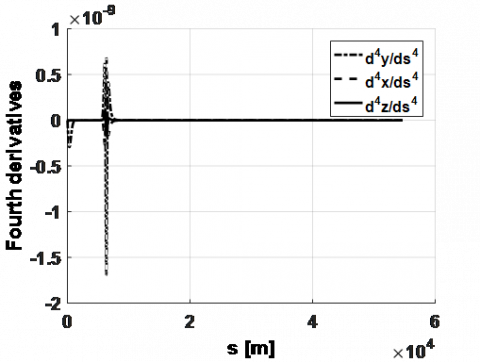Figure 6. Fourth derivatives of (x, y, z) with respect to trajectory path ## 7.pngFigure 7. Input to inverse simulation (overall trajectory) ## 8.pngFigure 8. Results of inverse simulation ## 9.png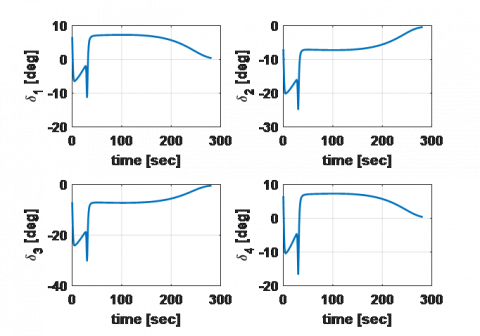Figure 9. Control surface deflection of the four fins ## 10.png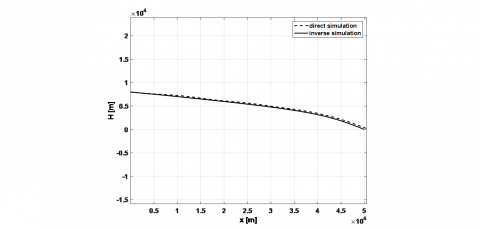Figure 10. Trajectory in vertical plane (comparison) The direct simulation of a glide trajectory is performed with a time step of ten milliseconds. A sudden raise in the fourth derivative of the trajectory position vector after the turning glide can be noticed (as shown in Figure 6). Therefore, the overall trajectory has some imperfections at the connection between the first two phases. ## 11.png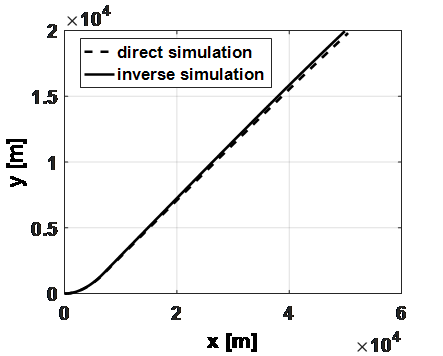Figure 11. Trajectory in horizontal plane (comparison) ## 12.pngFigure 12. Angle of attack response ## 13.png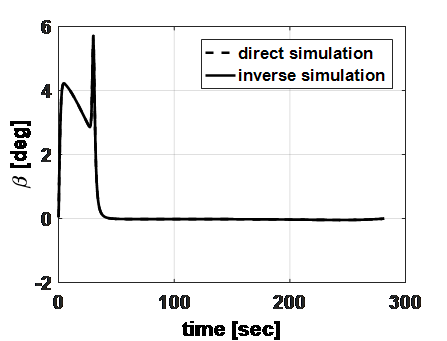Figure 13. Sideslip response ## 14.png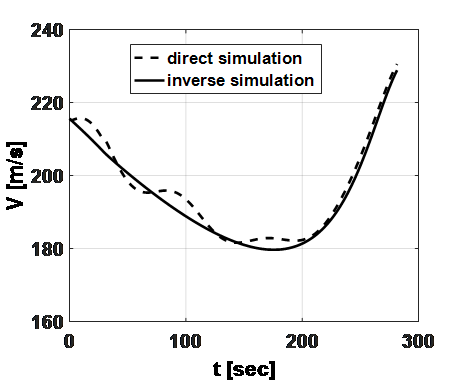Figure 14. Velocity response ## 15.pngFigure 15. Pitch angle response ## 16.png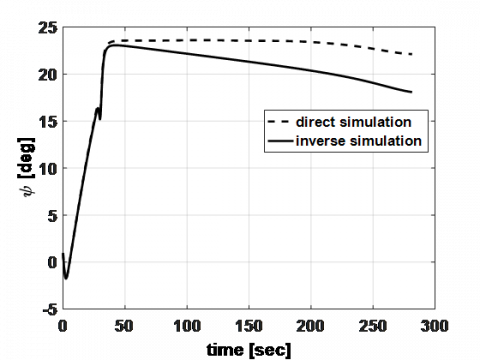Figure 16. Heading angle response ## 17.pngFigure 17. Bank angle response ## 18.pngFigure 18. Inverse and direct overall trajectories (comparisons) In order to validate the inverse simulation method, a direct simulation technique is performed using the deflection angles (similar to ) which are the output of the proposed method. A comparison between both the direct and the inverse simulations is then plotted (Figure 10 to Figure 18). The direct and inverse trajectories responses are matched. To demonstrate the accuracy of the method, the percentage of difference at the trajectory end point is calculated. The error in altitude, lateral displacement, angle of attack, sideslip angle, pitch angle, heading angle, and bank angle are found to be 2.5%, 1%, 0.02%, 0.869%, 1.1%, 0.033%, 16.6%, and 16.6%, respectively. 5. Conclusions In this paper, a new three-dimensional path planning and following tool for six degrees of freedom subsonic guided gliders has been developed. This tool generates an optimal trajectory depending on the required ground target impact angle and velocity. Despite the sensitivity to the trajectory generation imperfections, the proposed inverse simulation method results fairly match those of the direct simulation. These simulations involve the usage of Euler angles, which are more conveniently used in aeronautics (in contrast with quaternions). The proposed method involves pre-formulated inverse dynamics equations of motion which decreases the computational cost significantly. As an extension to the current work, a dual quaternion approach  could be used in order to increase the efficiency of the inverse simulation besides the decrease in the computational cost. The ongoing research includes the inverse and direct simulations in a closed loop control with a position feedback system, such as the GPS/INS system, to eliminate the effect of disturbances e.g. random wind effect. Nomenclature $C_{L}, C_{D,} C_{m}$Lift, drag, and pitching moment coefficients.$C_{Y}, C_{l}, C_{n}$Lateral force, rolling, and yawing moment coefficients.$C_{L o}, C_{D o}, C_{m o}$Lift, drag, and pitching moment coefficients at zero values of$\alpha$, q,$\delta$, and$\dot{\alpha}C_{Y o}, C_{l o}, C_{n o}$Lateral force, rolling, and yawing moment coefficients at zero values of$\beta$, p, r, and$\delta$.$C_{L \alpha}, C_{L q}, C_{L \delta_{p}}, C_{L \dot{\alpha}}$Derivatives of lift coefficient with respect to$\alpha$, q,$\delta_{p}$, and$\dot{\alpha}$.$C_{Y \beta}, C_{Y p}, C_{Y r}, C_{Y \delta_{r}}$Derivatives of lateral force coefficient with respect to$\beta$, p, r, and$\delta_{r}$.$C_{m \alpha}, C_{m q}, C_{m \delta_{p}}, C_{m \dot{\alpha}}$Derivatives of moment coefficient with respect to$\alpha$, q,$\delta_{p}$, and$\dot{\alpha}$.$C_{l \beta}, C_{l p}, C_{l r}, C_{l \delta_{r}}$Derivatives of rolling moment coefficient with respect to$\beta$, p, r, and$\delta_{r}$.$C_{n \beta}, C_{n p}, C_{n r}, C_{n \delta_{r}}$Derivatives of yawing moment coefficient with respect to$\beta$, p, r, and$\delta_{r}$.$p, q, r$Scalar components of angular velocity in body axis [rad/sec].$u, v, w$Scalar components of linear velocity in body axis [m/s].$\vec{V}_{B}$Velocity vector in body frame [m/s].$V_{t}$Total velocity [m/s].$x, y, z$Position vector in NED frame [m].$\alpha, \beta$Wind angles [deg].$\rho_{H}$_air Air density at (H) altitude [kg/m3].$\delta_{r}, \delta_{p}, \delta_{y}$Roll, pitch, and yaw fins deflection angles [deg].$\phi, \theta, \psi$Euler angles [deg].$\vec{\omega}_{B}\$ Angular velocity vector in body axis [rad/s].
Appendix

Equations of Attitudes Derivatives

theta_dot = -(calpha*cbeta*cgam*gam_dot ...

+ calpha*cgam*cmeu*alpha_dot - cbeta*salpha*sgam*alpha_dot ...

- calpha*sbeta*sgam*beta_dot - cmeu*salpha*sgam*gam_dot ...

- cgam*salpha*smeu*meu_dot + calpha*cbeta*cgam*smeu*beta_dot ...

+ calpha*cgam*cmeu*sbeta*meu_dot - cgam*salpha*sbeta*smeu*alpha_dot ...

- calpha*sbeta*sgam*smeu*gam_dot)/cth;

phi_dot=-(cbeta*sgam*beta_dot + cgam*sbeta*gam_dot ...

- sphi*sth*theta_dot ...

- cbeta*cgam*cmeu*meu_dot ...

+ cgam*sbeta*smeu*beta_dot + cbeta*sgam*smeu*gam_dot)/(cphi*cth);

psi_dot=-(salpha*(skai*smeu*kai_dot ...

- ckai*cmeu*meu_dot - sgam*skai*smeu*meu_dot ...

+ cgam*cmeu*skai*gam_dot + ...

ckai*cmeu*sgam*kai_dot) + ...

calpha*sbeta*(cgam*skai*smeu*gam_dot ...

- ckai*smeu*meu_dot - cmeu*skai*kai_dot ...

+ ckai*sgam*smeu*kai_dot + cmeu*sgam*skai*meu_dot) ...

- spsi*sth*theta_dot ...

- calpha*(ckai*smeu - cmeu*sgam*skai)*alpha_dot ...

+ calpha*cbeta*(ckai*cmeu + sgam*skai*smeu)*beta_dot ...

- salpha*sbeta*(ckai*cmeu + sgam*skai*smeu)*alpha_dot...

- calpha*cbeta*cgam*ckai*kai_dot ...

+ cbeta*cgam*salpha*skai*alpha_dot ...

+ calpha*cgam*sbeta*skai*beta_dot...

+ calpha*cbeta*sgam*skai*gam_dot)/(cpsi*cth);

theta_2dot = -(- sth*theta_dot^2 ...

- calpha*cbeta*sgam*alpha_dot^2 - calpha*cbeta*sgam*beta_dot^2 ...

- calpha*cbeta*sgam*gam_dot^2 + calpha*cbeta*cgam*gam_2dot...

- cgam*cmeu*salpha*alpha_dot^2 + calpha*cgam*cmeu* alpha_2dot ...

- cgam*cmeu*salpha* gam_dot^2 - cgam*cmeu*salpha* meu_dot^2 ...

- cbeta*salpha*sgam* alpha_2dot - calpha*sbeta*sgam* beta_2dot ...

- cmeu*salpha*sgam* gam_2dot - cgam*salpha*smeu* meu_2dot ...

- 2*cbeta*cgam*salpha* alpha_dot* gam_dot - calpha*cgam*sbeta*smeu* alpha_dot^2 ...

- 2*calpha*cgam*sbeta* gam_dot* beta_dot - calpha*cgam*sbeta*smeu* beta_dot^2 ...

+ calpha*cbeta*cgam*smeu* beta_2dot - calpha*cgam*sbeta*smeu* gam_dot^2 ...

- 2*calpha*cmeu*sgam* alpha_dot* gam_dot - calpha*cgam*sbeta*smeu* meu_dot^2 ...

+ calpha*cgam*cmeu*sbeta* meu_2dot - 2*calpha*cgam*smeu* alpha_dot* meu_dot ...

+ 2*salpha*sbeta*sgam* beta_dot* alpha_dot - cgam*salpha*sbeta*smeu* alpha_2dot ...

- calpha*sbeta*sgam*smeu* gam_2dot + 2*salpha*sgam*smeu* gam_dot* meu_dot ...

+ 2*calpha*cbeta*cgam*cmeu* beta_dot* meu_dot ...

- 2*cbeta*cgam*salpha*smeu* beta_dot* alpha_dot ...

- 2*calpha*cbeta*sgam*smeu* beta_dot* gam_dot ...

- 2*cgam*cmeu*salpha*sbeta* alpha_dot* meu_dot ...

- 2*calpha*cmeu*sbeta*sgam* gam_dot* meu_dot ...

+ 2*salpha*sbeta*sgam*smeu* alpha_dot* gam_dot)/cth;

phi_2dot =-(cbeta*sgam*beta_2dot ...

- sbeta*sgam*beta_dot^2 - sbeta*sgam*gam_dot^2 ...

+ cgam*sbeta*gam_2dot - cth*sphi*phi_dot^2 ...

- cth*sphi*theta_dot^2 ...

- sphi*sth*theta_2dot + 2*cbeta*cgam*beta_dot*gam_dot ...

+ cbeta*cgam*smeu*beta_dot^2 + cbeta*cgam*smeu*gam_dot^2 ...

+ cbeta*cgam*smeu*meu_dot^2 - cbeta*cgam*cmeu*meu_2dot ...

+ cgam*sbeta*smeu*beta_2dot + cbeta*sgam*smeu*gam_2dot ...

- 2*cphi*sth*phi_dot*theta_dot + 2*cgam*cmeu*sbeta*meu_dot*beta_dot ...

+ 2*cbeta*cmeu*sgam*gam_dot*meu_dot ...

- 2*sbeta*sgam*smeu*beta_dot*gam_dot)/(cphi*cth);

psi_2dot =-(salpha*(ckai*smeu*kai_dot^2 ...

+ ckai*smeu*meu_dot^2 - ckai*cmeu*meu_2dot ...

+ skai*smeu*kai_2dot - cmeu*sgam*skai*gam_dot^2 ...

- cmeu*sgam*skai*kai_dot^2 + cgam*cmeu*skai*gam_2dot ...

- cmeu*sgam*skai*meu_dot^2 + ckai*cmeu*sgam*kai_2dot ...

+ 2*cmeu*skai*meu_dot*kai_dot - sgam*skai*smeu*meu_2dot ...

+ 2*cgam*ckai*cmeu*kai_dot*gam_dot ...

- 2*cgam*skai*smeu*meu_dot*gam_dot ...

- 2*ckai*sgam*smeu*meu_dot*kai_dot) ...

- cth*spsi*theta_dot^2 - cth*spsi*psi_dot^2 ...

- spsi*sth*theta_2dot + salpha*(ckai*smeu ...

- cmeu*sgam*skai)*alpha_dot^2 - calpha*(ckai*smeu ...

- cmeu*sgam*skai)*alpha_2dot - calpha*sbeta*(ckai*cmeu*meu_dot^2 ...

+ cmeu*skai*kai_2dot + ckai*smeu*meu_2dot ...

+ ckai*cmeu*kai_dot^2 + sgam*skai*smeu*gam_dot^2 ...

+ sgam*skai*smeu*kai_dot^2 - cgam*skai*smeu*gam_2dot ...

+ sgam*skai*smeu*meu_dot^2 - ckai*sgam*smeu*kai_2dot ...

- cmeu*sgam*skai*meu_2dot - 2*skai*smeu*meu_dot*kai_dot ...

- 2*cgam*ckai*smeu*gam_dot*kai_dot ...

- 2*cgam*cmeu*skai*gam_dot*meu_dot ...

- 2*ckai*cmeu*sgam*kai_dot*meu_dot) ...

+ 2*calpha*(skai*smeu*kai_dot ...

- ckai*cmeu*meu_dot - sgam*skai*smeu*meu_dot ...

+ cgam*cmeu*skai*gam_dot + ckai*cmeu*sgam*kai_dot)*alpha_dot ...

+ 2*calpha*cbeta*(cgam*skai*smeu*gam_dot - ckai*smeu*meu_dot ...

- cmeu*skai*kai_dot + ckai*sgam*smeu*kai_dot ...

+ cmeu*sgam*skai*meu_dot)*beta_dot ...

- 2*salpha*sbeta*(cgam*skai*smeu*gam_dot ...

- ckai*smeu*meu_dot - cmeu*skai*kai_dot ...

+ ckai*sgam*smeu*kai_dot + cmeu*sgam*skai*meu_dot)*alpha_dot ...

- calpha*sbeta*(ckai*cmeu + sgam*skai*smeu)*alpha_dot^2 ...

- calpha*sbeta*(ckai*cmeu + sgam*skai*smeu)*beta_dot^2 ...

+ calpha*cbeta*(ckai*cmeu + sgam*skai*smeu)*beta_2dot ...

- 2*cpsi*sth*psi_dot*theta_dot - salpha*sbeta*(ckai*cmeu ...

+ sgam*skai*smeu)*alpha_2dot + calpha*cbeta*cgam*skai*alpha_dot^2 ...

+ calpha*cbeta*cgam*skai*beta_dot^2 ...

+ calpha*cbeta*cgam*skai*gam_dot^2 ...

+ calpha*cbeta*cgam*skai*kai_dot^2 ...

- calpha*cbeta*cgam*ckai*kai_2dot ...

+ cbeta*cgam*salpha*skai*alpha_2dot ...

+ calpha*cgam*sbeta*skai*beta_2dot ...

+ calpha*cbeta*sgam*skai*gam_2dot ...

- 2*cbeta*salpha*(ckai*cmeu + sgam*skai*smeu)*alpha_dot*beta_dot ...

+ 2*cbeta*cgam*ckai*salpha*kai_dot*alpha_dot ...

+ 2*calpha*cgam*ckai*sbeta*kai_dot*beta_dot ...

+ 2*calpha*cbeta*ckai*sgam*gam_dot*kai_dot ...

- 2*cgam*salpha*sbeta*skai*beta_dot*alpha_dot...

- 2*cbeta*salpha*sgam*skai*gam_dot*alpha_dot ...

- 2*calpha*sbeta*sgam*skai*beta_dot*gam_dot)/(cpsi*cth);

References

 Wang, G.G., Chu, H.E., Mirjalili, S. (2016). Three-dimensional path planning for UCAV using an improved bat algorithm. Aerospace Science and Technology, 49: 231-238. https://doi.org/10.1016/j.ast.2015.11.040

 Zhang, Y.L., Chen, K.J., Liu, L.H., Tang, G.J., Bao, W.M. (2016). Entry trajectory planning based on three-dimensional acceleration profile guidance. Aerospace Science and Technology, 48: 131-139. https://doi.org/10.1016/j.ast.2015.11.009

 Yao, P., Wang, H., Su, Z. (2015). Real-time path planning of unmanned aerial vehicle for target tracking and obstacle avoidance in complex dynamic environment. Aerospace Science and Technology, 47: 269-279. https://doi.org/10.1016/j.ast.2015.09.037

 Ma, Y., Guo, J., Tang, S. (2015). High angle of attack command generation technique and tracking control for agile missiles. Aerospace Science and Technology, 45: 324-334. https://doi.org/10.1016/j.ast.2015.06.003

 Feng, B.M., Nie, W.S. (2013). A new method for initial parameters optimization of guided projectiles. In Advanced Materials Research, 791: 1100-1104. https://doi.org/10.4028/www.scientific.net/AMR.791-793.1100

 Yang, L., Chen, W., Liu, X., Zhou, H. (2016). Steady glide dynamic modeling and trajectory optimization for high lift-to-drag ratio reentry vehicle. International Journal of Aerospace Engineering, 2016: 3527460. https://doi.org/10.1155/2016/3527460

 Zhang, D.C., Xia, Q.L., Wen, Q.Q., Zhou, G.Q. (2015). An approximate optimal maximum range guidance scheme for subsonic unpowered gliding vehicles. International Journal of Aerospace Engineering, 2015: 389751. https://doi.org/10.1155/2015/389751

 Kelley, H.J., Cliff, E.M., Lutze, F.H. (1982). Boost-glide range-optimal guidance. Optimal Control Applications and Methods, 3(3): 293-298. https://doi.org/10.1002/oca.4660030307

 Austin, S. (1998). Investigation of range extension with a genetic algorithm. In 7th AIAA/USAF/NASA/ISSMO Symposium on Multidisciplinary Analysis and Optimization, 1302-1310. https://doi.org/10.2514/6.1998-4880

 Vinh, N.X., Yang, C.Y., Chern, J.S. (1984). Optimal trajectories for maximum endurance gliding in a horizontal plane. Journal of Guidance, Control, and Dynamics, 7(2): 246-248. https://doi.org/10.2514/3.8575

 Lu, P. (2008). Entry trajectory optimization with analytical feedback bank angle law. AIAA Paper, 7268: 1-18. https://doi.org/10.2514/6.2008-7268

 Drury, R.G. (2010). Trajectory generation for autonomous unmanned aircraft using inverse dynamics. http://dspace.lib.cranfield.ac.uk/handle/1826/5583

 Drury, R.G., Tsourdos, A., Cooke, A.K. (2011). Negative-g trajectory generation using quaternion-based inverse dynamics. Journal of Guidance, Control, and Dynamics, 34(1): 283-287. https://doi.org/10.2514/1.51486

 Rahman, T., Hao, Z., Wanchun, C. (2013). Bézier approximation based inverse dynamic guidance for entry glide trajectory. In 2013 9th Asian Control Conference (ASCC), Istanbul, Turkey, pp. 1-6. https://doi.org/10.1109/ASCC.2013.6606111

 Yu, W., Chen, W. (2011). Guidance scheme for glide range maximization of a hypersonic vehicle. In AIAA Guidance, Navigation, and Control Conference, 6714. https://doi.org/10.2514/6.2011-6714

 Indig, N., Ben-Asher, J.Z., Farber, N. (2014). Near-optimal spatial midcourse guidance law with an angular constraint. Journal of Guidance, Control, and Dynamics, 37(1): 214-223. https://doi.org/10.2514/1.60356

 Huang, G.Q., Nan, Y., Chen, F., Wang, Y.T. (2009). Optimal Release Conditions of No-Power Gliding Bomb. Flight Dynamics, 27: 93-96.

 Hou, M.S. (2007). Optimal gliding guidance in vertical plane for standoff released guided bomb unit. Journal of China Ordnance, 5: 1-14.

 Darby, C., Rao, A. (2008). An initial examination of using pseudospectral methods for timescale and differential geometric analysis of nonlinear optimal control problems. In AIAA/AAS Astrodynamics Specialist Conference and Exhibit, 1-26. https://doi.org/10.2514/6.2008-6449

 Jorris, T.R., Cobb, R.G. (2009). Three-dimensional trajectory optimization satisfying waypoint and no-fly zone constraints. Journal of Guidance, Control, and Dynamics, 32(2): 551-572. https://doi.org/10.2514/1.37030

 Lu, P. (1993). Inverse dynamics approach to trajectory optimization for an aerospace plane. Journal of Guidance, Control, and Dynamics, 16(4): 726-732. https://doi.org/10.2514/3.21073

 Lou, K.Y., Bryson, A.E. (1996). Inverse and optimal control for precision aerobatic maneuvers. Journal of Guidance, Control, and Dynamics, 19(2): 483-488. https://doi.org/10.2514/3.21643

 Elsherbiny, A.M., Aly, A.M., Elshabka, A., Abdelrahman, M. (2018). Inverse simulation of symmetric flight of a guided gliding subsonic flying body. In 2018 AIAA Modeling and Simulation Technologies Conference, 0427. https://doi.org/10.2514/6.2018-0427

 Sentoh, E., Bryson, A.E. (1992). Inverse and optimal control for desired outputs. Journal of Guidance, Control, and Dynamics, 15(3): 687-691. https://doi.org/10.2514/3.20892

 Abdelrahman, M., Al-Bahi, A. (1994). A generalized technique for the inverse simulation of aircraft motion along predetermined trajectories. In 19th Atmospheric Flight Mechanics Conference, pp. 3523. https://doi.org/10.2514/6.1994-3523

 Blajer, W., Goszczyński, J.A., Krawczyk, M. (2002). The inverse simulation study of aircraft flight path reconstruction. Transport, 17(3): 103-107. https://doi.org/10.1080/16483840.2002.10414022

 Yang, Y., Yan, Y. (2016). Neural network approximation-based nonsingular terminal sliding mode control for trajectory tracking of robotic airships. Aerospace Science and Technology, 54: 192-197. https://doi.org/10.1016/j.ast.2016.04.021

 Yang, X., Wu, H., Li, Y., Kang, S., Chen, B. (2019). Computationally efficient inverse dynamics of a class of six-DOF parallel robots: Dual quaternion approach. Journal of Intelligent & Robotic Systems, 94(1): 101-113. https://doi.org/10.1007/s10846-018-0800-1

 Elsherbiny, A.M., Aly, A.M., Elshabka, A., Abdelrahman, M. (2018). Modeling, simulation and hyprid optimization method as design tools for range extension kit of a subsonic flying body. In 2018 AIAA Modeling and Simulation Technologies Conference, 0429. https://doi.org/10.2514/6.2018-0429

 Siouris, G.M. (2004). Missile guidance and control systems. Springer Science & Business Media.

 Kato, O., Sugiura, I. (1986). An interpretation of airplane general motion and control as inverse problem. Journal of Guidance, Control, and Dynamics, 9(2): 198-204. https://doi.org/10.2514/3.20090

 Miele, A. (2016). Flight mechanics: theory of flight paths. Courier Dover Publications.